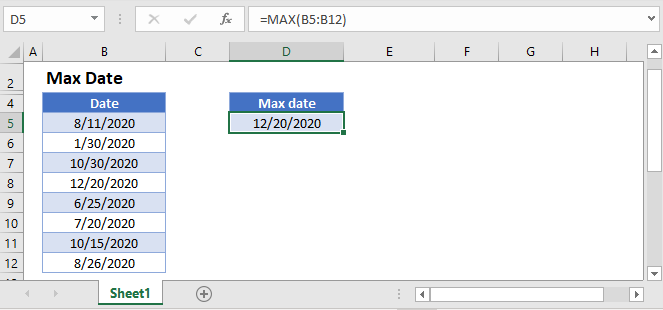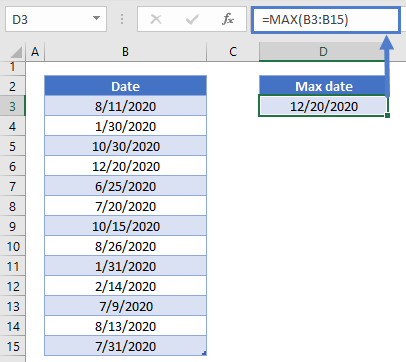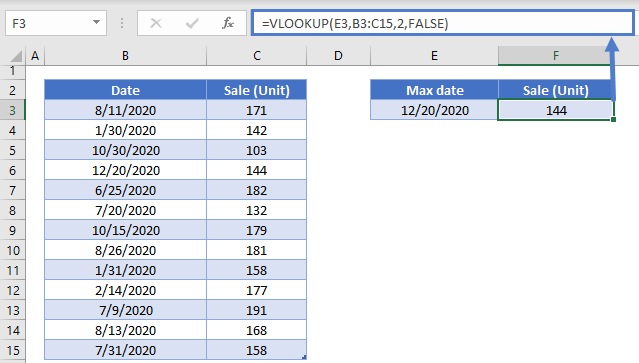# Max Date in Excel and Google Sheets

This tutorial will demonstrate how to calculate the max date in Excel and Google Sheets. It will also demonstrate how to lookup the max date in a list and return a corresponding value.## Calculate Max Date

You can use the MAX Function to calculate the max date in a list:

``=MAX(B3:B15)``### Max Date Lookup

After calculating the max date, you can perform a VLOOKUP (or XLOOKUP) to return a value corresponding to that date:

``=VLOOKUP(E3,B3:C15,2,FALSE)``### Max Date Sum

Alternatively, you can use the SUMIF Function to total all the values associated with that date from a list:

``=SUMIF(B3:B15,E3,C3:C15)``

## Google Sheets – Max Date

All of the above examples work exactly the same in Google Sheets as in Excel.### Excel Practice Worksheet

Practice Excel functions and formulas with our 100% free practice worksheets!